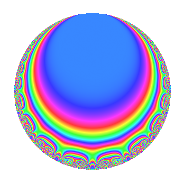# Properties

 Label 128.3.c.aLevel 128 Weight 3 Character orbit 128.c Analytic conductor 3.488 Analytic rank 0 Dimension 4 CM No Inner twists 2

# Related objects

## Newspace parameters

 Level: $$N$$ = $$128 = 2^{7}$$ Weight: $$k$$ = $$3$$ Character orbit: $$[\chi]$$ = 128.c (of order $$2$$ and degree $$1$$)

## Newform invariants

 Self dual: No Analytic conductor: $$3.48774738381$$ Analytic rank: $$0$$ Dimension: $$4$$ Coefficient field: $$\Q(\zeta_{8})$$ Coefficient ring: $$\Z[a_1, \ldots, a_{5}]$$ Coefficient ring index: $$2^{7}$$ Sato-Tate group: $\mathrm{SU}(2)[C_{2}]$

## $q$-expansion

Coefficients of the $$q$$-expansion are expressed in terms of a basis $$1,\beta_1,\beta_2,\beta_3$$ for the coefficient ring described below. We also show the integral $$q$$-expansion of the trace form.

 $$f(q)$$ $$=$$ $$q + \beta_{1} q^{3} + ( -2 - \beta_{2} ) q^{5} + ( -\beta_{1} - \beta_{3} ) q^{7} + ( -3 - 2 \beta_{2} ) q^{9} +O(q^{10})$$ $$q + \beta_{1} q^{3} + ( -2 - \beta_{2} ) q^{5} + ( -\beta_{1} - \beta_{3} ) q^{7} + ( -3 - 2 \beta_{2} ) q^{9} + ( \beta_{1} + 2 \beta_{3} ) q^{11} + ( 6 - \beta_{2} ) q^{13} + ( -7 \beta_{1} + \beta_{3} ) q^{15} + ( -2 - 2 \beta_{2} ) q^{17} + ( 3 \beta_{1} - 2 \beta_{3} ) q^{19} + 8 q^{21} + ( 5 \beta_{1} - 3 \beta_{3} ) q^{23} + ( 11 + 4 \beta_{2} ) q^{25} + ( -4 \beta_{1} + 2 \beta_{3} ) q^{27} + ( -34 - \beta_{2} ) q^{29} + ( 12 \beta_{1} + 4 \beta_{3} ) q^{31} + ( -4 + 2 \beta_{2} ) q^{33} -4 \beta_{3} q^{35} + ( -10 + 7 \beta_{2} ) q^{37} + ( \beta_{1} + \beta_{3} ) q^{39} + ( 2 + 4 \beta_{2} ) q^{41} + ( -13 \beta_{1} - 4 \beta_{3} ) q^{43} + ( 70 + 7 \beta_{2} ) q^{45} + ( -6 \beta_{1} + 2 \beta_{3} ) q^{47} + ( 1 + 8 \beta_{2} ) q^{49} + ( -12 \beta_{1} + 2 \beta_{3} ) q^{51} + ( 22 - \beta_{2} ) q^{53} + ( 7 \beta_{1} + 7 \beta_{3} ) q^{55} + ( -44 - 10 \beta_{2} ) q^{57} + 11 \beta_{1} q^{59} + ( -74 + 7 \beta_{2} ) q^{61} + ( -\beta_{1} - 9 \beta_{3} ) q^{63} + ( 20 - 4 \beta_{2} ) q^{65} + ( -9 \beta_{1} + 6 \beta_{3} ) q^{67} + ( -72 - 16 \beta_{2} ) q^{69} + ( -9 \beta_{1} - \beta_{3} ) q^{71} + ( 22 + 6 \beta_{2} ) q^{73} + ( 31 \beta_{1} - 4 \beta_{3} ) q^{75} + ( 88 - 16 \beta_{2} ) q^{77} + ( 22 \beta_{1} - 10 \beta_{3} ) q^{79} + ( 29 - 6 \beta_{2} ) q^{81} + ( 29 \beta_{1} + 8 \beta_{3} ) q^{83} + ( 68 + 6 \beta_{2} ) q^{85} + ( -39 \beta_{1} + \beta_{3} ) q^{87} + ( 54 - 10 \beta_{2} ) q^{89} + ( -8 \beta_{1} - 12 \beta_{3} ) q^{91} + ( -128 - 16 \beta_{2} ) q^{93} + ( -35 \beta_{1} - 3 \beta_{3} ) q^{95} + ( -82 - 10 \beta_{2} ) q^{97} + ( 15 \beta_{1} + 16 \beta_{3} ) q^{99} +O(q^{100})$$ $$\operatorname{Tr}(f)(q)$$ $$=$$ $$4q - 8q^{5} - 12q^{9} + O(q^{10})$$ $$4q - 8q^{5} - 12q^{9} + 24q^{13} - 8q^{17} + 32q^{21} + 44q^{25} - 136q^{29} - 16q^{33} - 40q^{37} + 8q^{41} + 280q^{45} + 4q^{49} + 88q^{53} - 176q^{57} - 296q^{61} + 80q^{65} - 288q^{69} + 88q^{73} + 352q^{77} + 116q^{81} + 272q^{85} + 216q^{89} - 512q^{93} - 328q^{97} + O(q^{100})$$

Basis of coefficient ring:

 $$\beta_{0}$$ $$=$$ $$1$$ $$\beta_{1}$$ $$=$$ $$2 \zeta_{8}^{3} + 2 \zeta_{8}^{2} + 2 \zeta_{8}$$ $$\beta_{2}$$ $$=$$ $$-4 \zeta_{8}^{3} + 4 \zeta_{8}$$ $$\beta_{3}$$ $$=$$ $$2 \zeta_{8}^{3} - 6 \zeta_{8}^{2} + 2 \zeta_{8}$$
 $$1$$ $$=$$ $$\beta_0$$ $$\zeta_{8}$$ $$=$$ $$($$$$\beta_{3} + 2 \beta_{2} + 3 \beta_{1}$$$$)/16$$ $$\zeta_{8}^{2}$$ $$=$$ $$($$$$-\beta_{3} + \beta_{1}$$$$)/8$$ $$\zeta_{8}^{3}$$ $$=$$ $$($$$$\beta_{3} - 2 \beta_{2} + 3 \beta_{1}$$$$)/16$$

## Character Values

We give the values of $$\chi$$ on generators for $$\left(\mathbb{Z}/128\mathbb{Z}\right)^\times$$.

 $$n$$ $$5$$ $$127$$ $$\chi(n)$$ $$1$$ $$-1$$

## Embeddings

For each embedding $$\iota_m$$ of the coefficient field, the values $$\iota_m(a_n)$$ are shown below.

For more information on an embedded modular form you can click on its label.

Label $$\iota_m(\nu)$$ $$a_{2}$$ $$a_{3}$$ $$a_{4}$$ $$a_{5}$$ $$a_{6}$$ $$a_{7}$$ $$a_{8}$$ $$a_{9}$$ $$a_{10}$$
127.1
 0.707107 − 0.707107i −0.707107 − 0.707107i −0.707107 + 0.707107i 0.707107 + 0.707107i
0 4.82843i 0 −7.65685 0 1.65685i 0 −14.3137 0
127.2 0 0.828427i 0 3.65685 0 9.65685i 0 8.31371 0
127.3 0 0.828427i 0 3.65685 0 9.65685i 0 8.31371 0
127.4 0 4.82843i 0 −7.65685 0 1.65685i 0 −14.3137 0
 $$n$$: e.g. 2-40 or 990-1000 Significant digits: Format: Complex embeddings Normalized embeddings Satake parameters Satake angles

## Inner twists

Char. orbit Parity Mult. Self Twist Proved
1.a Even 1 trivial yes
4.b Odd 1 yes

## Hecke kernels

This newform can be constructed as the kernel of the linear operator $$T_{5}^{2} + 4 T_{5} - 28$$ acting on $$S_{3}^{\mathrm{new}}(128, [\chi])$$.